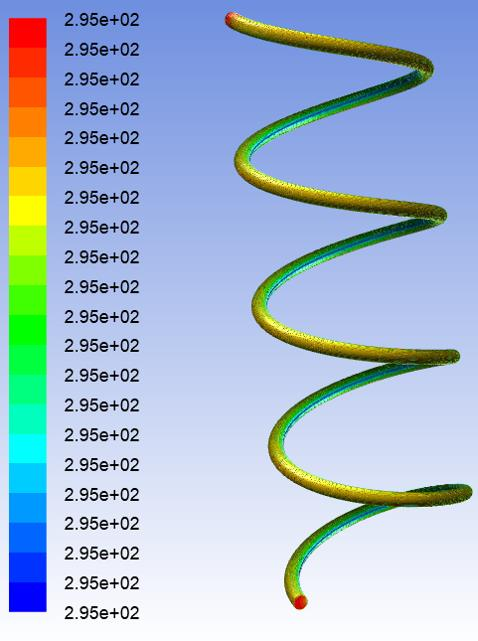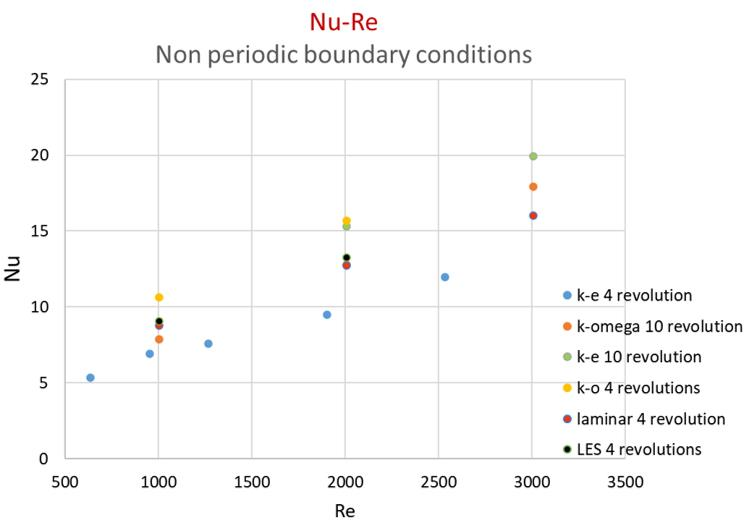# Average Nusselt number calculation for a helical tube using different viscous models

Hi all,

I am trying to explore the Nu-Re correlation of a helical tube. Constant wall temperature BC was used. I didn't set reference temperature to get Nu since I found that in this way Nu varied significantly with reference temperature. Instead, area weighted average heat flux and LMTD were usded to get heat transfer coefficient and then Nu. LMTD was calculated by the area weighted average temperature at the inlet and outlet, and the constant wall temperature.

Now the problem is why the Nu sometimes increases when I use longer tube (10 revolutions other than 4 revolutions). I have tried K-epsilon, k-omega, LES and laminar models.

Another problem is that I found that when periodic BC was used, the fluid temperature would converge to the wall temperature no matter how large the difference betweeen the upstream temperature and wall temperature I set. Then both the heat flux along the wall and LMTD are very small, under this condition, how to calculate the Nu?